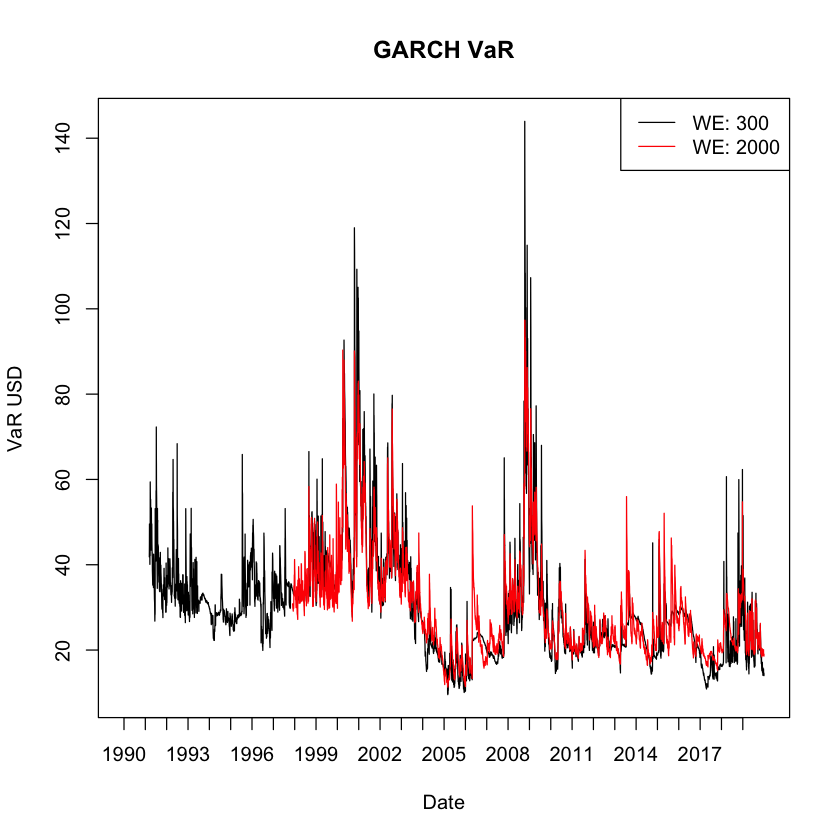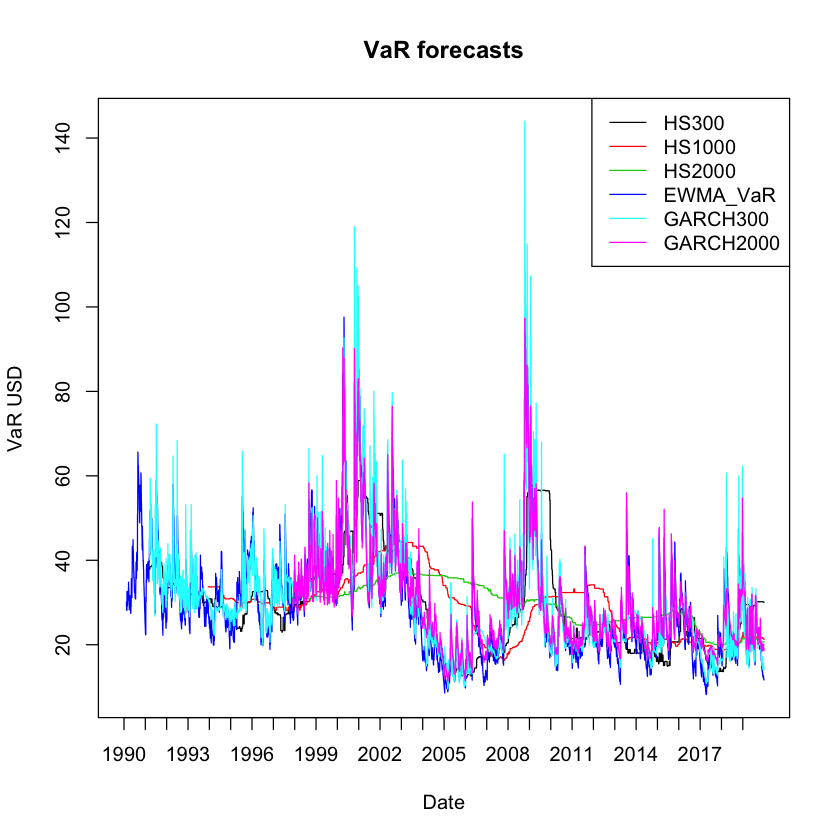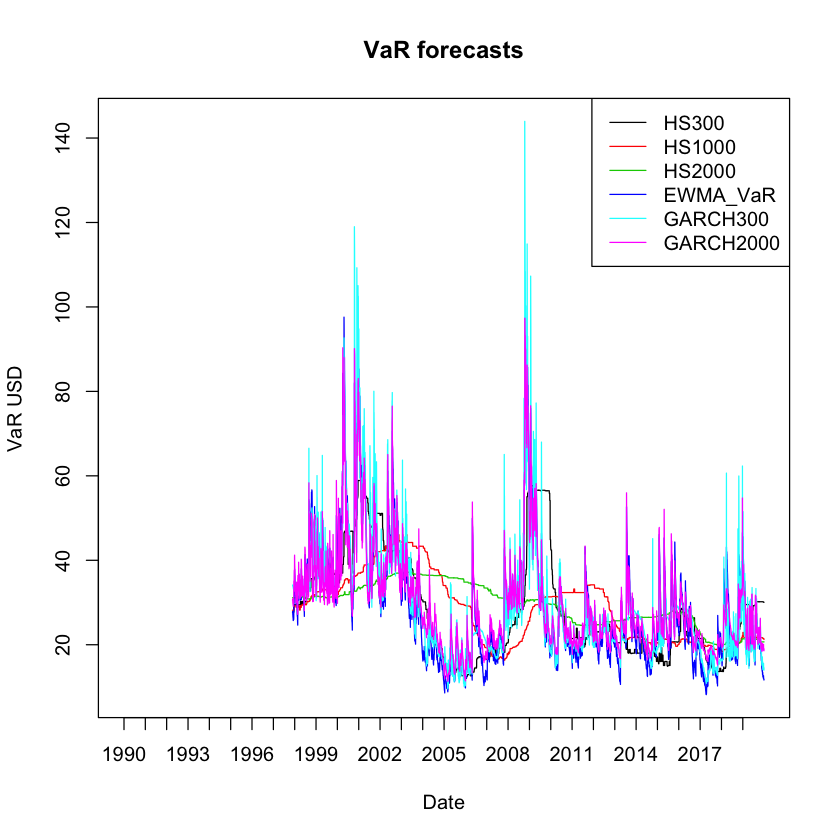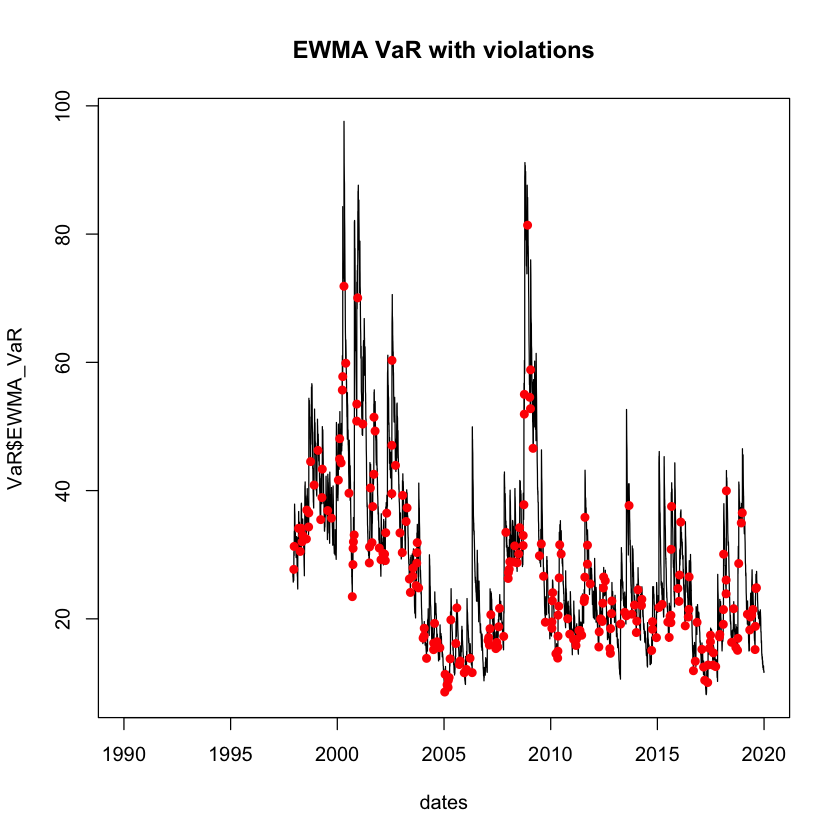#### FM442: Quantitative Methods for Finance and Risk Analysis

Jon Danielsson
Michaelmas Term 2021

# Seminar 7

In this class we will:

We first load the packages for this seminar:

In :
library(lubridate)
library(rugarch)


We will continue building VaR forecasts for the returns of Microsoft. You should load the VaR data frame from the previous seminar, which should contain the columns:

• HS300
• HS1000
• HS2000
• EWMA_VaR
In :
# Load files from the previous seminar

y <- Y$MSFT dates <- Y$date

# Parameters
portfolio_value = 1000
p = 0.05


## GARCH VaR¶

To implement VaR forecast using GARCH for a given estimation window, we need to recursively fit the specified GARCH model to the moving estimation window to get the optimal parameters, and use these to forecast conditional volatility for the following day. Once we have the conditional volatility, we estimate VaR using the inverse cdf for the specified probability and the portfolio value. For example, if the estimation window is 1000 days, we should:

1. Use data from day 1 to day 1000 to fit the GARCH model
2. Extract the optimal coefficients
3. Find $\hat{\sigma}_{t+1}$ using the GARCH equation
4. Compute VaR by: $\textrm{VaR}_{t+1}(p) = -\hat{\sigma}_{t+1} F_{R}^{-1}(p)\ \vartheta$

Note that we will be fitting thousands of GARCH models, so this will take a long time to run.

We want to forecast GARCH VaR using estimations windows of 300 and 2000 days. The best way to do so is to write a function:

In [ ]:
# Steps inside the function:
# Take as argument
# GARCH spec, here the default
# Probability, here 0.05
# Portfolio value, here 1000
# Estimation window, here 1000
spec <- ugarchspec()
p <- 0.05
portfolio_value <- 1000
WE <- 1000

# Determine number of observations
n <- length(y)

# Initialize empty VaR vector

VaR <- rep(NA, n)

# Do a loop for the forecast
for (i in 1:(n-WE)){

# Subset the dataset to the estimation window
window <- y[i:(i+WE-1)]

# Fit the GARCH
res <- ugarchfit(spec = spec, data = window, solver = "hybrid")

# Save coefficients
omega <- coef(res)['omega']
alpha <- coef(res)['alpha1']
beta <- coef(res)['beta1']

# Estimate sigma2 using the last observation of window
sigma2 <- omega + alpha*tail(window,1)^2 + beta*tail(res@fit$var,1) # Allocate the VaR forecast in the vector VaR[i+WE] <- -sqrt(sigma2) * qnorm(probability) * portfolio_value }  Now let's put everything inside a function: In [ ]: # Function that creates a GARCH forecast DoGARCH <- function(y, spec, probability = 0.05, portfolio_value = 1, WE = 1000){ # GARCH function that takes as argument: # y: A vector of returns, ordered by date # spec: The ugarchspec object with the GARCH specification # probability: The probability to be used for VaR - Default 5% # portfolio_value: The portfolio value - Default 1 # WE: Estimation window for the forecast - Default 1000 days # To calculate elapsed time, first get the current time old <- Sys.time() # Print message cat("Doing GARCH VaR forecast", "\n", "Estimation window:", WE, "\n", "Number of observations:", length(y), "\n", "VaR probability:", probability, "\n", "Portfolio value:", portfolio_value) # Number of observations n <- length(y) # Initialize empty VaR vector VaR <- rep(NA, n) # Do a loop for the forecast for (i in 1:(n-WE)){ # Subset the dataset to the estimation window window <- y[i:(i+WE-1)] # Fit the GARCH res <- ugarchfit(spec = spec, data = window, solver = "hybrid") # Save coefficients omega <- coef(res)['omega'] alpha <- coef(res)['alpha1'] beta <- coef(res)['beta1'] # Estimate sigma2 using the last observation of window sigma2 <- omega + alpha*tail(window,1)^2 + beta*tail(res@fit$var,1)

# Allocate the VaR forecast in the vector
VaR[i+WE] <- -sqrt(sigma2) * qnorm(probability) * portfolio_value
}

# Get the new time and print the elapsed time
time <- difftime(Sys.time(), old, units = "secs")
cat("\n", "Elapsed time:", round(time,4), "seconds")

# Return the VaR vector
return(VaR)
}


Doing the GARCH VaR forecast for estimation windows of 300 and 2000 days:

In :
# Create specification
spec <- ugarchspec(
variance.model = list(garchOrder= c(1,1)),
mean.model= list(armaOrder = c(0,0), include.mean=FALSE)
)

In :
# GARCH VaR for 300 days
GARCH300 <- DoGARCH(y, spec = spec, probability = 0.05, portfolio_value = 1000, WE = 300)

Doing GARCH VaR forecast
Estimation window: 300
Number of observations: 7559
VaR probability: 0.05
Portfolio value: 1000
Elapsed time: 374.7003 seconds

Since the code takes a long time to run, we can save the output to avoid having to run it again:

In :
# Saving the output
save(GARCH300, file = "GARCH300.RData")

In :
# GARCH VaR for 2000 days
GARCH2000 <- DoGARCH(y, spec = spec, probability = 0.05, portfolio_value = 1000, WE = 2000)

Doing GARCH VaR forecast
Estimation window: 2000
Number of observations: 7559
VaR probability: 0.05
Portfolio value: 1000
Elapsed time: 631.4514 seconds
In :
# Saving the output
save(GARCH2000, file = "GARCH2000.RData")

In :
# If we have it already saved, we can load it by:


We can plot them together:

In :
# Bind into a matrix
GARCH_VaR <- cbind(GARCH300, GARCH2000)

# Plot and modify axis to include dates
matplot(dates, GARCH_VaR, type = "l", lty = 1, col = 1:2, xaxt = "n", main = "GARCH VaR", xlab = "Date", ylab = "VaR USD")
axis.Date(1, at = seq(min(dates), max(dates), by = "years"))

# Legend
legend("topright", legend = c("WE: 300", "WE: 2000"), lty = 1, col = 1:2)## Analyze and compare VaR forecasts¶

We now have 6 different VaR forecasts:

1. EWMA using WE = 30 days
2. HS using WE = 300 days
3. HS using WE = 1000 days
4. HS using WE = 2000 days
5. GARCH using WE = 300 days
6. GARCH using WE = 2000 days

In order to analyze and compare them, let's put them together in a matrix:

In :
# Creating a matrix for all VaR forecasts
VaR <- cbind(VaR, GARCH300, GARCH2000)


First, let's compare the means and standard deviation for each forecast. To get the standard deviation of each column, we can use the apply(), which takes as input a matrix, a function to be applied to each row/column, and a number: 1 for rows and 2 for columns.

Note that our models have different estimation windows, so the comparison is not completely fair because the number of NA is inconsistent accross the columns. We need to use the option na.rm = TRUE:

In :
# Means for each forecast
round(colMeans(VaR, na.rm = TRUE),3)

# Standard deviations - We
round(apply(VaR, 2, sd, na.rm = TRUE))

HS300
28.88
HS1000
28.844
HS2000
29.399
EWMA_VaR
29.988
GARCH300
30.193
GARCH2000
29.62
HS300
11
HS1000
8
HS2000
5
EWMA_VaR
13
GARCH300
13
GARCH2000
12

First, we see the mean is quite similar for all models. However, the standard deviations are quite different. We see that for the Historical Simulation models, a larger estimation window implies a smaller standard deviation of the forecasts. For the GARCH model, the effect of the size of the estimation window on the standard deviation of forecasts is not clear.

Let's use matplot() to plot the six forecasts:

In :
# Plot all
matplot(dates, VaR, type = "l", lty = 1, col = 1:6, xaxt = "n", main = "VaR forecasts", xlab = "Date", ylab = "VaR USD")
axis.Date(1, at = seq(min(dates), max(dates), by = "years"))

# Legend
legend("topright", legend = colnames(VaR), lty = 1, col = 1:6)Since all our models have different estimation windows, we focus on the most restrictive one:

In :
# Find maximum estimation window
windows <- colSums(is.na(VaR))

# Restrict to largest estimation window
VaR[1:max(windows),] <- NA

# Plot all
matplot(dates, VaR, type = "l", lty = 1, col = 1:6, xaxt = "n", main = "VaR forecasts", xlab = "Date", ylab = "VaR USD")
axis.Date(1, at = seq(min(dates), max(dates), by = "years"))

# Legend
legend("topright", legend = colnames(VaR), lty = 1, col = 1:6)## Backtesting¶

### Violation Rations¶

In order the forecasts of our different models, we will perform a backtest. This involves comparing the forecasted VaR for time $t$ to the realized return for that same date. If the return in time $t$ is a loss larger than the forecasted VaR, we count this as a violation:

VaR violation: an event such that $$\eta _{t} = \begin{cases} 1 & \text{if y_t ≤ -VaR_t} \\ 0 & \text{if y_t > -VaR_t}\\ \end{cases}$$

We proceed to count the violations in the Testing Window $W_T$, which is the length of the dataset minus the Estimation Window:

Violations: $v_1$
Non-violations: $v_0$

Where:

$$v_1 = \sum_{t=1}^{W_T} \eta_t$$$$v_0 = W_T - v_1$$

The main tool we use for backtesting are the violation rations. We compare the observed number of VaR violations with the expected number. If we are calculating a 5% VaR, we expect to see $5\% \times W_T$ violations.

We define the Violation Ratio as:

$$\text{VR} = \frac{\text{Observed violations}}{\text{Expected violations}} = \frac{v_1}{p\times W_T}$$

If the violation ratio is greater than one, it means we observe more violations than we expect, so our VaR model is underforecasting risk. On the other hand, if it is smaller than one, we are overforecasting risk.

We expect $\text{VR}=1$, but how can we interpret the $\text{VR}$ from a model? A useful rule of thumb is:

• If $\text{VR} \in [0.8,1.2]$ the model is good
• If $\text{VR} \in [0.5,0.8]\ \text{or}\ \text{VR} \in [1.2,1.5]$ the model is acceptable
• If $\text{VR} \in [0.3,0.5]\ \text{or}\ \text{VR} \in [1.5,2]$ the model is bad
• If $\text{VR} < 0.5\ \text{or}\ \text{VR} > 2$ the model is useless

### Backtesting in R¶

We will now calculate the Violation Ratios for each of our models:

In :
# Backtesting and Violation Ratios

# Let's transform VaR to a data.frame
VaR <- as.data.frame(VaR)

# Initialize a Violations data.frame, same dim and colnames as VaR, fill with NA
Violations <- VaR
Violations[] <- NA

dim(Violations)

1. 7559
2. 6

We are going to populate the Violations matrix using a loop that compares each day's VaR forecast for every model with the realized returns on that day. This is done with a logical, which is a type of data in R that returns TRUE or FALSE depending on the statement. Numerically, TRUE is equivalent to 1, and FALSE to 0. For example:

In :
# Logicals in R
a <- 10000 < 5
b <- 10000 > 5

a
b

sum(a,b)

FALSE
TRUE
1

So we will compare each value in VaR with the realized return:

In :
# Populating the Violations matrix

# For every model (columns in VaR)
for(i in 1:dim(VaR)){

# Fill the column in Violations with TRUE/FALSE
# TRUE if the realized return is lower than VaR
# FALSE otherwise
Violations[,i] <- y*portfolio_value < -VaR[,i]
}


We can use the which() function to see where the Violations happened in every model:

In :
# Find where violations happened
dates[which(Violations$EWMA_VaR)]  Let's pick one of the days where HS2000 is violated and see if the other models also violate VaR: In : # Get a random day where EWMA VaR is violated using sample() random_day <- sample(dates[which(Violations$HS2000)],1)

# Find the index in dates using which()
day_index <- which(dates == random_day)

# See that row in Violations
paste0("Violation for HS2000 on ",random_day)
Violations[day_index,]

'Violation for HS2000 on 2011-08-10'
HS300HS1000HS2000EWMA_VaRGARCH300GARCH2000
5448TRUETRUETRUETRUETRUETRUE

We can easily plot the VaR forecasts and add points where the Violations happened using the points() function.

A useful feature about logical vectors is that we can use them to subset other objects. For example:

In :
# Subsetting with logical vectors
logi <- c(TRUE, FALSE, TRUE, TRUE)
vec <- c(1,2,3,4)

# If we subset by "logi", we will only keeps positions where TRUE
vec[logi]

1. 1
2. 3
3. 4

We can use this feature to subset our objects to where violations happened:

In :
# Plotting the violations
plot(dates, VaR$EWMA_VaR, type = "l", main = "EWMA VaR with violations") # Add points where the violations happened points(dates[Violations$EWMA_VaR], VaR$EWMA_VaR[Violations$EWMA_VaR], pch = 16, col = "red")Using the & operator, let's see if they are dates for which all our models have a VaR violation. We can use apply() and the all() function, which checks if all elements are TRUE:

In :
# Check dates where all models have a violation
w <- apply(Violations, 1, all)


We can use sum() to cound the number of days where all models have a violation:

In :
# Days where all models have a violation
sum(w, na.rm = TRUE)

122

Let's plot these days with the returns:

In :
# Plotting the returns and adding the days where all models had a violation
plot(dates, y, main = "Microsoft returns", type = "l", lwd = 2, las = 1,
xlab = "Date", ylab = "Returns")
points(dates[w], y[w], pch = 16, col = "red")